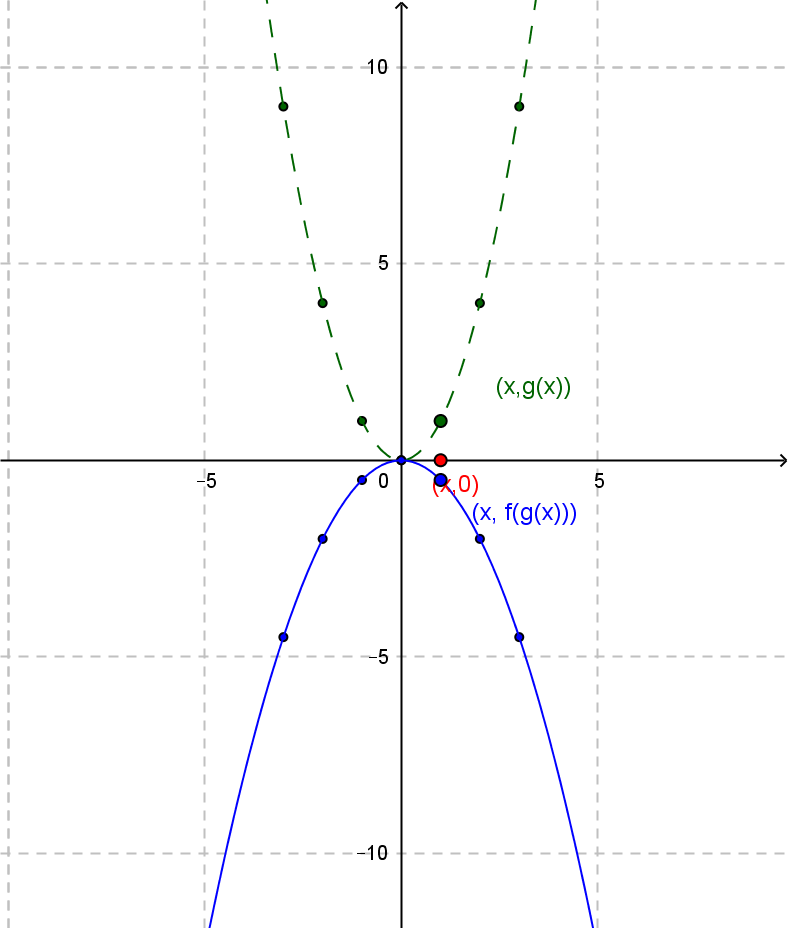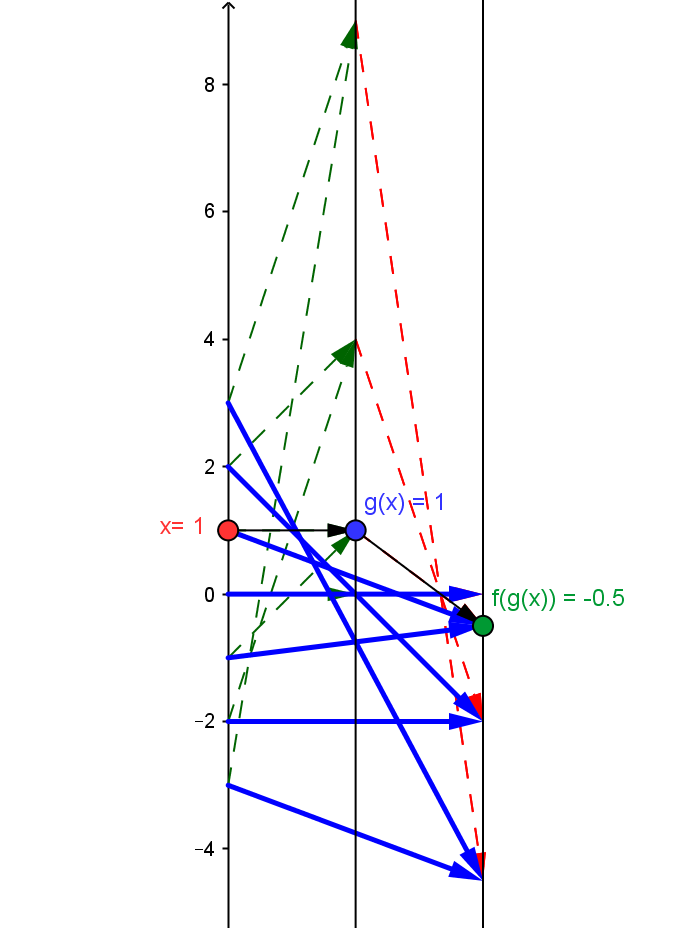Example QF.2.4.0 : $A =-\frac 1 2; q(x) = - \frac1 2 x^2$
This is best understood as the composition of the core quadratic function $g(x)= x^2$ followed by the linear function $f(x) =- \frac 1 2 x$, so $q(x) = f(g(x))$.
Draw a mapping diagram yourself or  use the diagram created with GeoGebra to explore the diagram further.
Compare the mapping diagram with the graphs of $g(x)$ and $q(x)$
 Graphs of $g(x)$ and $q(x)$Mapping Diagram Showing Composition. Given a point / number, $x$, on the source line, there is a blue arrow meeting the target line at the point / number, $-\frac 1 2 x^2$. This point corresponds to the quadratic function's value for $x$. The values for the core mapping diagram for $x^2$ in green are magnified by a factor of $-\frac 1 2$  shown by the red arrows.As $x$ increases, $q(x)$ increases to value $q(0)=0$ and then decreases.